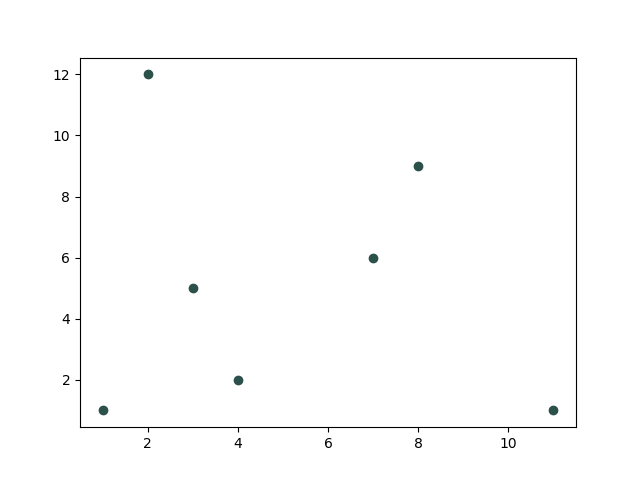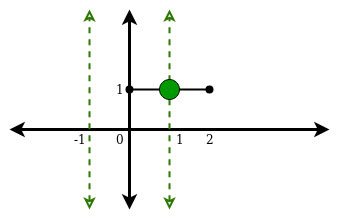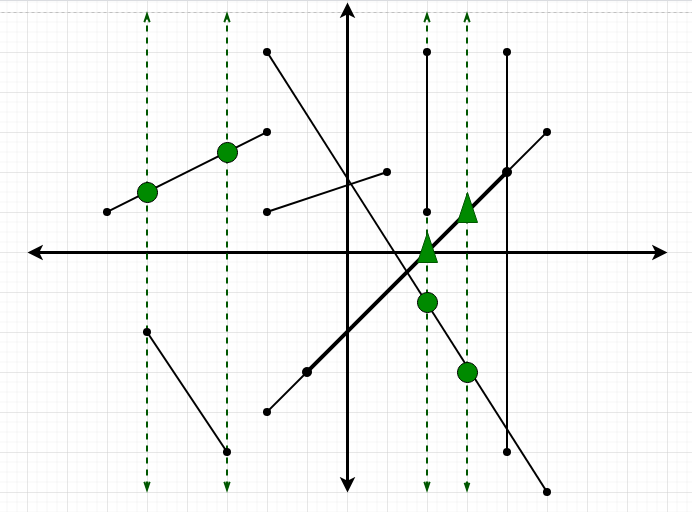1598712720

# The Geometric Median

Given a set of points in the plane, where can we place another point C such that the sum of the distances from C to the other points is minimized? This is the problem that we’ll attempt to solve in this article.

To visualize, consider the following set of points:Input points: (1,1), (3,5), (4,2), (7,6), (8,9), (11,1), (2,12)

The problem looks somewhat similar to the centroid (the point that minimizes the sum of the squared distances to the other points). Although we can find formulas in the case of the centroid, it is impossible to do the same for the geometric median, and so, the only way to approach the latter is by finding a numerical approximation.#mathematics #computer-science #numerical-methods #optimization #geometry #data science

## Buddha Community1597348800

## Find the point on X-axis from given N points having least Sum of Distances

Given an array **arr[] **consisting of **N **integers, denoting N points lying on the X-axis, the task is to find the point which has the least sum of distances from the all other points.

Example:

Input:_ arr[] = {4, 1, 5, 10, 2}_

Output:_ (4, 0)_

Explanation:

Distance of 4 from rest of the elements = |4 – 1| + |4 – 5| + |4 – 10| + |4 – 2| = 12

Distance of 1 from rest of the elements = |1 – 4| + |1 – 5| + |1 – 10| + |1 – 2| = 17

Distance of 5 from rest of the elements = |5 – 1| + |5 – 4| + |5 – 2| + |5 – 10| = 13

Distance of 10 from rest of the elements = |10 – 1| + |10 – 2| + |10 – 5| + |10 – 4| = 28

Distance of 2 from rest of the elements = |2 – 1| + |2 – 4| + |2 – 5| + |2 – 10| = 14

Input:_ arr[] = {3, 5, 7, 10}_

Output:_ 5_

Naive Approach:

The task is to iterate over the array, and for each array element, calculate the sum of its absolute difference with all other array elements. Finally, print the array element with the maximum sum of differences.

Time Complexity:_ O(N2)_

Auxiliary Space:_ O(1)_

**Efficient Approach: **To optimize the above approach, the idea is to find the median of the array. The median of the array will have the least possible total distance from other elements in the array. For an array with even number of elements, there are two possible medians and both will have the same total distance, return the one with the lower index since it is closer to origin.

Follow the below steps to solve the problem:

• Sort the given array.
• If **N **is odd, return the **(N + 1 / 2)th **element.
• Otherwise, return the **(N / 2)th **element.

Below is the implementation of the above approach:

• C++

`// C++ Program to implement`

`// the above approach`

`#include <bits/stdc++.h>`

`**using**` `**namespace**` `std;`

`// Function to find median of the array`

`**int**` `findLeastDist(``**int**` `A[],` `**int**` `N)`

`{`

`// Sort the given array`

`sort(A, A + N);`

`// If number of elements are even`

`**if**` `(N % 2 == 0) {`

`// Return the first median`

`**return**` `A[(N - 1) / 2];`

`}`

`// Otherwise`

`**else**` `{`

`**return**` `A[N / 2];`

`}`

`}`

`// Driver Code`

`**int**` `main()`

`{`

`**int**` `A[] = { 4, 1, 5, 10, 2 };`

`**int**` `N =` `**sizeof**``(A) /` `**sizeof**``(A);`

`cout <<` `"("` `<< findLeastDist(A, N)`

`<<` `", "` `<< 0 <<` `")"``;`

`**return**` `0;`

`}`

Output:

``````(4, 0)
``````

Time Complexity:_ O(Nlog(N))_

Auxiliary Space:_ O(1)_

Attention reader! Don’t stop learning now. Get hold of all the important DSA concepts with the DSA Self Paced Course at a student-friendly price and become industry ready.

#arrays #geometric #mathematical #sorting #geometric-lines #median-finding1624608788

## How to Find Mean, Median, and Mode in Python?

Mean, median, and mode are fundamental topics of statistics. You can easily calculate them in Python, with and without the use of external libraries.

These three are the main measures of central tendency. The central tendency lets us know the “normal” or “average” values of a dataset. If you’re just starting with data science, this is the right tutorial for you.By the end of this tutorial you’ll:

• Understand the concept of mean, median, and mode
• Be able to create your own mean, median, and mode functions in Python
• Make use of Python’s statistics module to quickstart the use of these measurements

If you want a downloadable version of the following exercises, feel free to check out the GitHub repository.

Let’s get into the different ways to calculate mean, median, and mode.

#development #python #how to find mean, median, and mode in python #find mean #median #mode1623422100

## Introduction

In a series of weekly articles, I will be covering some important topics of statistics with a twist.

The goal is to use Python to help us get intuition on complex concepts, empirically test theoretical proofs, or build algorithms from scratch. In this series, you will find articles covering topics such as random variables, sampling distributions, confidence intervals, significance tests, and more.

At the end of each article, you can find exercises to test your knowledge. The solutions will be shared in the article of the following week.

Articles published so far:

As usual, the code is available on my GitHub.

#math #machine-learning #python #statistics #geometric and poisson random variables with python #geometric and poisson1597341600

## Minimum Sum of Euclidean Distances to all given Points

Given a matrix mat[][] consisting of N pairs of the form {x, y} each denoting coordinates of N points, the task is to find the minimum sum of the Euclidean distances to all points.

Examples:

_Input: _mat[][] = { { 0, 1}, { 1, 0 }, { 1, 2 }, { 2, 1 }}

Output: 4

Explanation:

Average of the set of points, i.e. Centroid = ((0+1+1+2)/4, (1+0+2+1)/4) = (1, 1).

Euclidean distance of each point from the centroid are {1, 1, 1, 1}

Sum of all distances = 1 + 1 + 1 + 1 = 4

Input:_ mat[][] = { { 1, 1}, { 3, 3 }}_

_Output: _2.82843

### Approach:

Since the task is to minimize the Euclidean Distance to all points, the idea is to calculate the Median of all the pointsGeometric Median generalizes the concept of median to higher dimensions

Follow the steps below to solve the problem:

• Calculate the centroid of all the given coordinates, by getting the average of the points.
• Find the Euclidean distance of all points from the centroid.
• Calculate the sum of these distance and print as the answer.

Below is the implementation of above approach:

• C++

`// C++ Program to implement`

`// the above approach`

`#include <bits/stdc++.h>`

`**using**` `**namespace**` `std;`

`// Function to calculate Euclidean distance`

`**double**` `find(``**double**` `x,` `**double**` `y,`

`vector<vector<``**int**``> >& p)`

`{`

`**double**` `mind = 0;`

`**for**` `(``**int**` `i = 0; i < p.size(); i++) {`

`**double**` `a = p[i], b = p[i];`

`mind +=` `**sqrt**``((x - a) * (x - a)`

`+ (y - b) * (y - b));`

`}`

`**return**` `mind;`

`}`

`// Function to calculate the minimum sum`

`// of the euclidean distances to all points`

`**double**` `getMinDistSum(vector<vector<``**int**``> >& p)`

`{`

`// Calculate the centroid`

`**double**` `x = 0, y = 0;`

`**for**` `(``**int**` `i = 0; i < p.size(); i++) {`

`x += p[i];`

`y += p[i];`

`}`

`x = x / p.size();`

`y = y / p.size();`

`// Calculate distance of all`

`// points`

`**double**` `mind = find(x, y, p);`

`**return**` `mind;`

`}`

`// Driver Code`

`**int**` `main()`

`{`

`// Initializing the points`

`vector<vector<``**int**``> > vec`

`= { { 0, 1 }, { 1, 0 }, { 1, 2 }, { 2, 1 } };`

`**double**` `d = getMinDistSum(vec);`

`cout << d << endl;`

`**return**` `0;`

`}`

Output:

``````4
``````

Time Complexity:_ O(N)_

_Auxiliary Space: _O(1)

Attention reader! Don’t stop learning now. Get hold of all the important DSA concepts with the DSA Self Paced Course at a student-friendly price and become industry ready.

#arrays #geometric #mathematical #median-finding #code1594564500

## Count of intersections of M line segments with N vertical lines in XY plane

Given x coordinates of N vertical lines (parallel to Y-axis) and M line segments extending from (x1, y1) to (x2, y2), the task is to find the total number of intersections of the line segments with the vertical lines.

Examples:

Input:_ N = 2, M = 1, lines[] = {-1, 1}, Segments[] = {0, 1, 2, 1}_

Output:_ 1_

Explanation:

There is only one point of intersection (1, 1)Input:_ N = 4, M = 8, lines[] = {-5, -3, 2, 3}, segments[] = {{-2, 5, 5, -6}, {-5, -2, -3, -5}, {-2, 3, -6, 1}, {-1, -3, 4, 2}, { 2, 5, 2, 1}, { 4, 5, 4, -5}, {-2, -4, 5, 3}, { 1, 2, -2, 1}};_

Output:_ 8_

Explanation:

There are total of 8 intersections.

Dotted lines are the vertical lines.

A green circle denote a single point of intersection and

a green triangle denotes that two line segments

intersect same vertical line at that point.Naive Approach:

The simplest approach is, for each query, check if a vertical line falls between the x-coordinates of the two points. Thus, each segment will have O(N) computational complexity.

Time complexity:_ O(N * M)_

Approach 2: The idea is to use Prefix Sum to solve this problem efficiently. Follow the steps below to solve the problem:

• The first observation we can make is that the y-coordinates do not matter. Also, we can observe that just touching the vertical line does not count as an intersection.
• First, compute a prefix array of the number of occurrences of vertical lines till now and then just subtract the number of occurrences till x2-1 (we don’t consider x2 as it just qualifies as touch and not as an intersection) from the number of occurrences till x1. So for each segment, computational complexity reduces to O(1).

Below is the implementation of the above approach.

#arrays #competitive programming #geometric #hash #cpp-map #geometric-lines